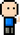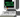# Josh Meets Computer# Knapsack Problem

October 21, 2018

''' Problem Description: You are a cake thief with a bag that has a weight capacity of N. You are in a shop with K cakes, each cake has a weight W and a price C.

Figure out the combination of cakes that give you the most value for what you can fit in your bag.

Assumptions And Constraints:

• 0 < k < 1000 ~ a limited number of types of cake

• You can steal as many cakes from one type as you want

• 0 < N < 100 ~ a limited amount of weight in your bag

• 0 < W < 1000000 ~ a lot of weight options

• 0 < N < 1000000 ~ a lot of price options

• Is the cake list in any particular order?

• How long is the cake list?

Intuition: search problem, looking for optimal solution space (subset of cakes) for each cake weight: determine max value you can get from each cake if there is remaining capacity, determine max value from remaining capacity

Examples: Inputs: N = 20 cake_list = [(3, 90), (7, 200), (2, 40)]

20 / 3 = 6; 20 % 3 = 2; 2 / 2 = 1; 90 * 6(units of 3) + 40 * 1(units of 2) = 580

20 / 7 = 2; 20 % 7 = 6; 6 / 3 = 2, 6 / 2 = 3 200 * 2(units of 7) + 90 * 3

20 |—divide by 7, 6 remain {0 + 200 * 2 = 400} 6 remain |—divide by 3, 0 remain {400 + 90 * 2 = 580} |—divide by 2, 0 remain {400 + 40 * 3 = 620} <— max |—divide by 3, 2 remain {0 + 90 * 6 = 540} 2 remain |—divide by 2, 0 remain {540 + 40 * 1 = 580} |—divide by 2, 0 remain {0 + 40 * 10 = 400}

Output: max_value = 620 '''

``````def calc_cakes(N, cake_list):
"""
Approach:
For each possible capacity between 1 and N, calculate the maximum
value stored for that weight.
The maximum value is determined by:
max (N / cake_weight for each cake_weight * cake_value)
+ max(N % cake_weight)
Args:
N (int): the weight of the bag
cake_list (list of tuples): weight and price of each cake

Returns:
max_value = maximum value of cakes you fit in your bag

"""

MAX_DOLLARS =  * (N+1)

for capacity in range(1, N+1):
possible_cakes = [(w, v) for (w, v) in cake_list if w <= c]

max_value = 0
remaining_capacity = 0
for w, v in possible_cakes:
cake_value = capacity // w * v

if cake_value > max_value:
max_value = cake_value
remaining_capacity = capacity % w

# update the maximum value for that capacity
MAX_DOLLARS[capacity] = max_value + MAX_DOLLARS[remaining_capacity]

return MAX_DOLLARS[N+1]

def calc_value(N, cake_list, curr_val)
"""
Approach:
Calculates the max value, returns curr_value. This takes a greedy approach.
Divide capacity N by each cake weight, keeping the cake which has the max
value. Repeat with remaining capacity until you can't fit any more cakes or there is no more room

Args:
N (int): the weight of the bag
cake_list (list of tuples): weight and price of each cake
curr_val (int): monetary value that can be held
Returns:
max_value = maximum value of cakes you fit in your bag
"""
if N == 0:
return curr_val
max_val = 0
remaining_capacity = None
for w, v in cake_list:
if (N / w) * v > max_val:  # 380 > 0
max_val = (N / w) * v  # 380
remaining_capacity = N % w  # 6
return calc_value(remaining_capacity, cake_list, max_val + curr_val)  # (6, cl, 400) + (0, cl, 180), (2, cl, 540) + (0, cl, 40),  (0, cl, 400)``````